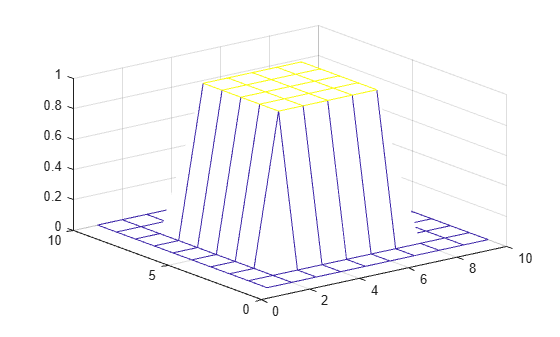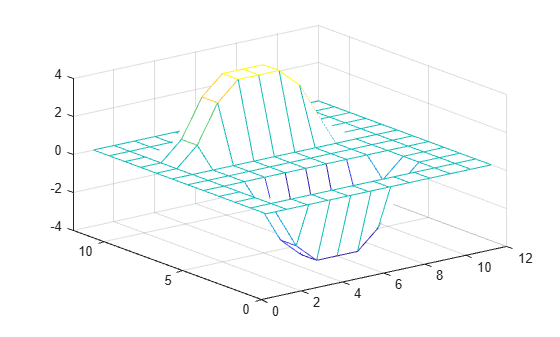# filter2

2-D digital filter

## Syntax

``Y = filter2(H,X)``
``Y = filter2(H,X,shape)``

## Description

example

````Y = filter2(H,X)` applies a finite impulse response filter to a matrix of data `X` according to coefficients in a matrix `H`.```

example

````Y = filter2(H,X,shape)` returns a subsection of the filtered data according to `shape`. For example, `Y = filter2(H,X,'valid')` returns only filtered data computed without zero-padded edges.```

## Examples

collapse all

You can digitally filter images and other 2-D data using the `filter2` function, which is closely related to the `conv2` function.

Create and plot a 2-D pedestal with interior height equal to one.

```A = zeros(10); A(3:7,3:7) = ones(5); mesh(A)```Filter the data in `A` according to a filter coefficient matrix `H`, and return the full matrix of filtered data.

```H = [1 2 1; 0 0 0; -1 -2 -1]; Y = filter2(H,A,'full'); mesh(Y)```Rotate `H` 180 degrees and convolve the result with `A`. The output is equivalent to filtering the data in `A` with the coefficients in `H`.

```C = conv2(A,rot90(H,2)); mesh(C)```## Input Arguments

collapse all

Coefficients of the rational transfer function, specified as a matrix.

Data Types: `double` | `single` | `int8` | `int16` | `int32` | `int64` | `uint8` | `uint16` | `uint32` | `uint64` | `logical`
Complex Number Support: Yes

Input data, specified as a matrix. If one or both of `X` and `H` are of type `single`, then the output is also of type `single`. Otherwise, `filter2` returns type `double`.

Data Types: `double` | `single` | `int8` | `int16` | `int32` | `int64` | `uint8` | `uint16` | `uint32` | `uint64` | `logical`
Complex Number Support: Yes

Subsection of the filtered data, specified as one of these values:

• `'same'` — Return the central part of the filtered data, which is the same size as `X`.

• `'full'` — Return the full 2-D filtered data.

• `'valid'` — Return only parts of the filtered data that are computed without zero-padded edges.

## Algorithms

The `filter2` function filters data by taking the 2-D convolution of the input `X` and the coefficient matrix `H` rotated 180 degrees. Specifically, `filter2(H,X,shape)` is equivalent to `conv2(X,rot90(H,2),shape)`.

## Version History

Introduced before R2006a